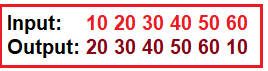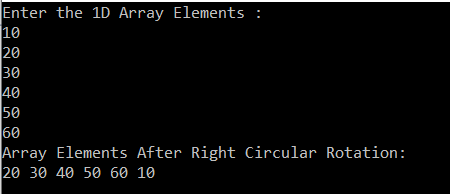# How to Perform Left Circular Rotation of an Array in C#

## How to Perform Left Circular Rotation of an Array in C#

In this article, I am going to discuss How to Perform Left Circular Rotation of an Array in C# with an example. Please read our previous article where we discussed how to convert a one-dimensional array to a two-dimensional array in C# with an example.

##### Program Description:

Here, the user will input an integer array. Then we need to shift each element of the input array to its left by one position in the circular fashion. The logic we need to implement should iterate the loop from Length – 1 to 0 and then swap each element with the last element. Please have a look at the following diagram for a better understanding of what we want to achieve.##### Left Circular Rotation of an Array in C#:

In the following example, first we take the input integer array from the user. Then we perform the left circular rotation using for loop.

```using System;
namespace LogicalPrograms
{
class Program
{
static void Main(string[] args)
{
int[] oneDimensionalArray = new int;
Console.WriteLine("Enter the 1D Array Elements : ");
for (int i = 0; i<oneDimensionalArray.Length; i++)
{
}

int temp;
for (int j = 0; j < oneDimensionalArray.Length - 1; j++)
{
temp = oneDimensionalArray;
oneDimensionalArray = oneDimensionalArray[j + 1];
oneDimensionalArray[j + 1] = temp;
}

Console.WriteLine("Array Elements After Right Circular Rotation: ");
foreach (int num in oneDimensionalArray)
{
Console.Write(num + " ");
}

}
}
}

```

Output:In the next article, I am going to discuss how to perform the Right circular rotation of an array in C# with examples. I hope now you understood How to Perform Left Circular Rotation of an Array in C#.

### 6 thoughts on “How to Perform Left Circular Rotation of an Array in C#”

1.the logic is wrong
for (int j = 0; j < oneDimensionalArray.Length – 1; j++)
{
temp = oneDimensionalArray;
oneDimensionalArray = oneDimensionalArray[j + 1];
oneDimensionalArray[j + 1] = temp;
}

it prints 60,10,20,30,40,50

1.Shashikant kumar

just change the 0 value of index to j like
temp = oneDimensionalArray[j];
oneDimensionalArray[j] = oneDimensionalArray[j + 1];
oneDimensionalArray[j + 1] = temp;

2.Hi
In one of the interview I was given task of reversing the elements of matrix with out using temp variable how can we write that can you please write the solution in logical program’s in C#
[3, 1, 5, 8, 4] is orginal array
1st task output should be [1, 3, 5, 8, 4]
2nd task output should be [1, 8, 5, 3, 4]

this should be done without temp variable

1.just write it as a[i]=a[n-i-1]

3.Here is the Program : While taking input from the user, just store the 1st element in a temporary List, and later add that temp element at the end of the output array.

Console.WriteLine(“Please enter the length of the array”);
List new_lst= new List();
int[] out_arr = new int[a];

for (int i = 0; i < a; i++)
{
if (i == 0)
{

}
else
{

}

}
foreach(var i in new_lst)
{
out_arr[a – 1] = i;
}

return out_arr ;

4.Hi,